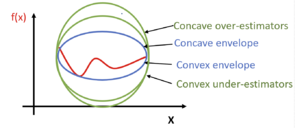# McCormick envelopes: Difference between revisions

Author: Susan Urban (smu29) (SYSEN 5800 Fall 2021)

## Introduction

Optimization of a non-convex function f(x) is challenging since it may have multiple locally optimal solutions or no solution and it can take a significant amount of time, computing resources, and effort to determine if the solution is global or the problem has no feasible solution. Different techniques are used to address this challenge depending on the characteristics of the problem.Figure 1: Relationships between the given function, concave over-estimators, convex under-estimators, a convex envelope, and a concave envelope.

One technique used for a given non-convex function is the identification of a concave envelope and a convex envelope. The concave envelope, and respectively the convex envelope, is the concave over-estimator and convex under-estimator for the given function providing the tighest fit to the given function. The envelope surrounds the given function, like an envelope encloses a letter, and limits the feasible solution space the most in comparison to all other concave over-estimators and convex under-estimators. Multiple concave over-estimators and multiple convex under-estimators may exist but there is only one concave envelope and one convex envelope for a given function and domain.

## McCormick Envelopes: Theory, Methodology and Algorithmic Discussions

The McCormick Envelope, originally developed by Garth McCormick1, is a type of convex relaxation used for the optimization of bilinear (e.g., x*y, x+y) non-linear programming (NLP) problems.

Each bilinear term is replaced with a new variable and four sets of constraints are added. The non-linear programming is converted to a relaxed convex linear programming (LP) which can be more easily solved.

The LP solution gives a lower (L) bound and any feasible solution to the problem gives an upper (U) bound.

As noted by Scott et al2, McCormick envelopes are effective since they are recursive, can be applied to a variety of applications, and are typically stronger than those resulting from convexification or linearization procedures.

The following is a derivation of the McCormick Envelopes:

$Let\ w=xy$$x^{L}\leq x\leq x^{U}$$y^{L}\leq y\leq y^{U}$where $x^{L},x^{U},y^{L},y^{U}$are   upper  and   lower  bound  values  for  $x$and $y$, respectively.

$a=\left(x-x^{L}\right)$$b=\left(y-y^{L}\right)$$a*b\geq 0$$a*b=\left(x-x^{L}\right)\left(y-y^{L}\right)=xy-x^{L}y-xy^{L}+x^{L}y^{L}\geq 0$$w\geq x^{L}y+xy^{L}-x^{L}y^{L}$$a=\left(x^{U}-x\right)$$b=\left(y^{U}-y\right)$$w\geq x^{U}y+xy^{U}-x^{U}y^{U}$$a=\left(x^{U}-x\right)$$b=\left(y-y^{L}\right)$$w\leq x^{U}y+xy^{L}-x^{U}y^{L}$$a=\left(x-x^{L}\right)$$b=\left(y^{U}-y\right)$$w\leq xy^{U}+x^{L}y-x^{L}y^{U}$The under-estimators of the function are represented by:

$w\geq x^{L}y+xy^{L}-x^{L}y^{L}$$w\geq x^{U}y+xy^{U}-x^{U}y^{U}$The over-estimators of the function are represented by:

$w\leq x^{U}y+xy^{L}-x^{U}y^{L}$$w\leq xy^{U}+x^{L}y-x^{L}y^{U}$The following shows the relaxation of a non-convex problem:

Original non-convex problem:

$\min Z=\textstyle \sum _{i=1}\sum _{j=1}c_{i,j}x_{i}x_{j}+g_{0}(x)$$s.t.\sum _{i=1}\sum _{j=1}c_{i,j}^{l}x_{i}x_{j}+g_{l}(x)\leq 0,\forall l\in L$$x^{L}\leq x\leq x^{U}$Replacing $u_{i,j}=x_{i}x_{j}$we obtain a relaxed, convex problem:

$\min Z=\textstyle \sum _{i=1}\sum _{j=1}c_{i,j}u_{i,j}+g_{0}(x)$$s.t.\sum _{i=1}\sum _{j=1}c_{i,j}^{l}u_{i,j}+g_{l}(x)\leq 0,\forall l\in L$$u_{i,j}\geq x_{i}^{L}x_{j}+x_{i}x_{j}^{L}-{x_{i}}^{L}{x_{j}}^{L},\forall i,j\$$u_{i,j}\geq x_{i}^{U}x_{j}+x_{i}x_{j}^{U}-{x_{i}}^{U}{x_{j}}^{U},\forall i,j\$$u_{i,j}\geq x_{i}^{L}x_{j}+x_{i}x_{j}^{U}-{x_{i}}^{L}{x_{j}}^{U},\forall i,j\$$u_{i,j}\geq x_{i}^{U}x_{j}+x_{i}x_{j}^{L}-{x_{i}}^{U}{x_{j}}^{L},\forall i,j\$$x^{L}\leq x\leq x^{U},\ \ u^{L}\leq u\leq u^{U}$Good lower and upper bounds focus and minimize the feasible solution space; they reduce the number of iterations to find the optimal solution.

As discussed by Hazaji3, global optimization solvers focus initially on optimizing the lower and upper bounds, and when necessary, focus on domain partitioning. By dividing the domain of a given variable into partitions or smaller regions, the solver is able to tailor and further tighten the convex relaxations of each partition.

## Example: Numerical

$min\ Z=xy+6x+y$$s.t.\ xy\leq 18$$0\leq x\leq 10$$0\leq y\leq 2$$Let\ w=xy$$min\ Z=-w+6x+y$$s.t.\ w\leq 18$$w\geq 0$$w\geq 10y+2x-20$$w\leq 10y$$w\leq 2x$Using GAMS, the solution is z= -24, x=6, y=2.

GAMS code sample:

variable z;

positive variable x, y, w;

equations  obj, c1, c2, c3, c4, c5 ;

obj..    z =e= -w -2*x ;

c1..     w =l= 12 ;

c2..     w =g= 0;

c3..     w =g= 6*y +3*x -18;

c4..     w =l= 6*y;

c5..     w =l= 3*x;

x.up = 10;

x.lo =0;

y.up = 2;

y.lo = 0;

model course5800 /all/;

option mip = baron;

option optcr = 0;

solve course5800 minimizing z using mip ;

## Application

Bilinear functions occur in numerous engineering and natural science applications where McCormick Envelopes can be utilized, including the following :

Computer vision 4

Super resolution imaging 5

Energy conversion networks 6

Cellular networks7

Dynamic biological systems8

## Conclusion

Non-convex NLPs are challenging to solve and may require a significant amount of time, computing resources, and effort to determine if the solution is global or the problem has no feasible solution. McCormick Envelopes provide a relaxation technique for bilinear non-convex nonlinear programming problems. McCormick Envelopes provide a straightforward technique of replacing each bilinear term with a new variable and adding four constraints. Due to the recursive nature of this technique, it may be applied to a wide variety of engineering and scientific applications involving bilinear terms.